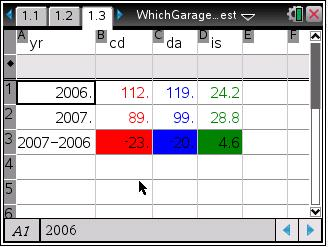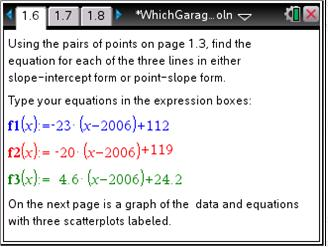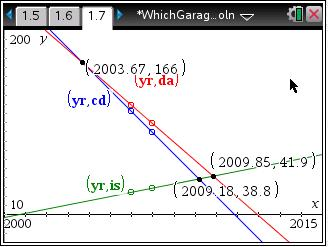### Mathematics lessons for IB® Diploma Programme

Applications and Interpretation | Functions

# Which Garage Is Better?

Students are to find the solution to a system of linear equations using technology. They are presented with two problems; music sales and parking lot fees.

## Key Steps## Step 1

In Problem 1, students are given data from music sales in a Lists & Spreadsheet page. They use this scenario to find the solution to a system of linear equations.

They use the cells to calculate the slope. Students use the expression = b2b1 in cell B3. They may see that since there is only a difference of 1 year, the difference between the sales data for CDs, digital albums, and individual songs is equal to the slope of the line.## Step 2

Students use the pairs of points for each format of music and find the equations for each of the three lines. These equations can be put in either slope-intercept form or point-slope form.## Step 3

Students graph the equations formed. After graphing them, they use the Intersection Point(s) tool to find where the lines cross each other. Students will see that there are 3 intersections points.

In doing this, they will see that sales of CDs will continue to go down but will not go away and in the latter part of 2009 the sales of individual songs will overtake the digital albums.